## A dipole encounters a monopole

#### monopole weaker / encounter head-on

A Lamb dipole with radius 0.75 and velocity of +2 (i.e. moving to the right) is placed to the left of a positive Bessel monopole with radius 0.50 at the origin.

• The circulation of the monopole is +4, which makes the monopole weaker than each dipole half, since the circulation per dipole half is about 10.2.
• The dipole is located at the x-axis: the encounter is head-on.
The initial situation is shown in the following two graphs: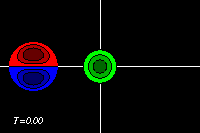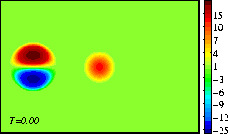Initial tracer (left) and vorticity (right) distribution;
passive tracers are placed along some streamlines.
The domain shown is x=[-3.0:+3.0], y=[-2.0:+2.0].
The extrema of voriticity are +/- 29.5 for the dipole
and +11.8 for the monopole.
The monopole has an angular momentum: it rotates about its central point, without moving. The dipole has a linear momentum: it moves in the direction of its axis, in this case to the right, towards the (weaker) monopole.

The monopole rotates counter-clockwise, like the top half of the dipole. The result of the rotation is that the dipole is pushed down, to negative y-values, while it moves towards the monopole. This can be seen clearest in the tracer plots: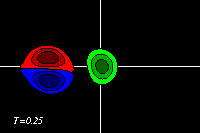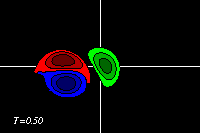Due to the approach of the dipole, the monopole is deformed and pushed away from its initial location. The dipole half is stronger than the monopole and because of that the dipole half pulls the monopole apart and around it: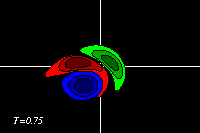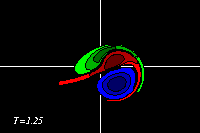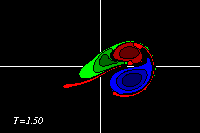The monopole clearly also disturbs the top half of the dipole: that half is deformed, and part of its positive (red) vorticiy is swept around the negative (blue) half of the dipole in the proces.

Both the top half of the dipole and the monopole are positive, so they can join and form one vorticity patch -- the two vorticity patches merge: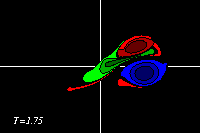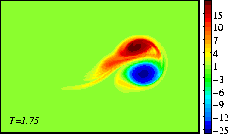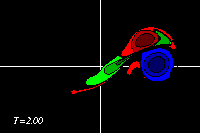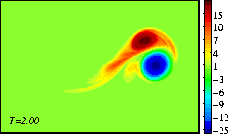At the moment of the last picture, the monopole is merged into the top half of the original dipole, making that half stronger. The result is thus a dipolar vortex which is a-symmetric: it will move along a curved path, with the stronger half on the inside. The merger is not complete: some of the vorticity of the monopole is left behind as a tail of positive vorticity.

These MPEG movies (81 frames; 456kb) show the evolution until T=2 more clearly:
===> MPEG movie of the tracer distribution
===> MPEG movie of the vorticity distribution

At initialisation, a single tracer particle was placed at the extrema of vorticity of both vortices. The next graph shows their trajectories:Trajectories of the tracers, initially placed at
the extrema of vorticity of the monopole (solid
line) and the dipole (dashed line).
Symbols are placed at intervals of T=0.25.

Outcome of the interaction

The outcome of the interaction depends on the value of several variables in the experiment, notably:
• the relative strengths
• the relative sizes
• the vertical offset -- see below
• and, to a lesser extend, the horizontal distance
of the vortices at initialisation.
The vertical offset is the vertical distance between the dipole's axis and the monopole's centre, which is often nambed b In the above example this offset is zero: b=0.

Consider a change in this vertical offset:
• b>>1:
If the dipole axis is far enough below the monopole's centre, it is clear that the monopole will deflect the dipole downward and the dipole will not meet the monopole: the dipole will pass the monopole.
• b<<-1:
If the dipole axis is far enough above the monopole's centre, the dipole will still move to negative y-values due to the monopole's rotation, but dipole will pass the monopole.
• intermediate cases:
In intermediate cases the encounter can lead to a merger as shown above or to an exchange of partners.
The latter means that the positive monopole joins up with the negative half of the dipole to form a new (a-symmetric) dipole that moves away, leaving the old positive dipole half behind as a monopole.
If the dipole is strong, then this "exchange of partners" can even be only temporarily.
What actually happens and at what value for b one mode switches to another depends on the other variables mentioned.

Three other examples are on these pages:
• a monopole weaker than each dipole half, as on this page, but with an encounter with an offset: b=+0.5, leading to a temporal "exchange of partners".
• a monopole nearly as strong as each dipole have, with the encounter:
• with an offset: b=+0.5, leading to a lasting "exchange of partners".

Viscous effects

Viscosity is included in the numerical experiment shown above.
The voricity levels decrease in time due to this viscosity, but on the short time scale of this experiment the decrease is negligible (less than 5%).
Viscosity is essential in the process though, as it is viscosity which makes the actual merger of the dipole half and the monopole into one single vortex possible: viscosity evens out the vorticity into one patch.

Negative monopole

If in the above example the monopole had been negative, it would have rotated clockwise and the monopole would have merged with the negative half of the dipole: the situation would be mirrored in the y-axis.

The evolution of the vorticity distribution is computed with a Finite Difference Method which solves the two-dimensional vorticity (Navier-Stokes) equation. Time and distances are given in dimensionless units.

===> Some details on the computation presented on this page for those who are interested.